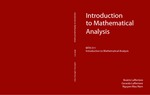# Material Detail## Introduction to Mathematical Analysis

Our goal with this textbook is to provide students with a strong foundation in mathematical analysis. Such a foundation is crucial for future study of deeper topics of analysis. Students should be familiar with most of the concepts presented here after completing the calculus sequence. However, these concepts will be reinforced through rigorous proofs. The textbook contain topics of real analysis usually covered in a 10-week course: the completeness axiom, sequences and convergence, continuity, and differentiation. The lecture notes also contain many well-selected exercises of various levels. Although these topics are written in a more abstract way compared with those available in some textbooks, teachers can choose to simplify them depending on the background of the students. For instance, rather than introducing the topology of the real line to students, related topological concepts can be replaced by more familiar concepts such as open and closed intervals. Some other topics such as lower and upper semicontinuity, differentiation of convex functions, and generalized differentiation of non-differentiable convex functions can be used as optional mathematical projects. In this way, the lecture notes are suitable for teaching students of different backgrounds.
Rate

#### Quality

• User Rating
• Learning Exercises
• Bookmark Collections
• Course ePortfolios
• Accessibility Info

#### Browse...

##### People who viewed this also viewed
••••##### Other materials like Introduction to Mathematical Analysis
••••